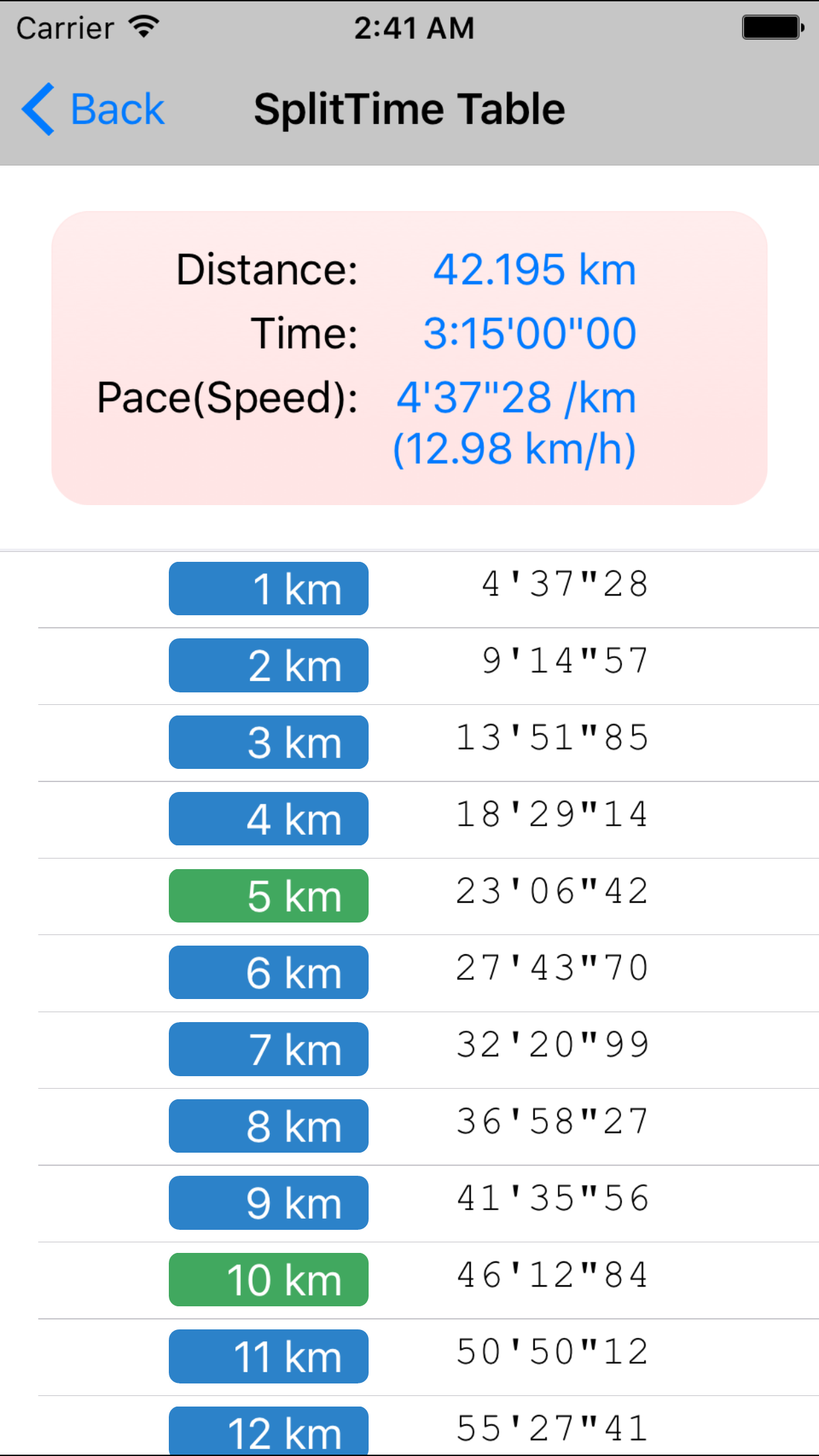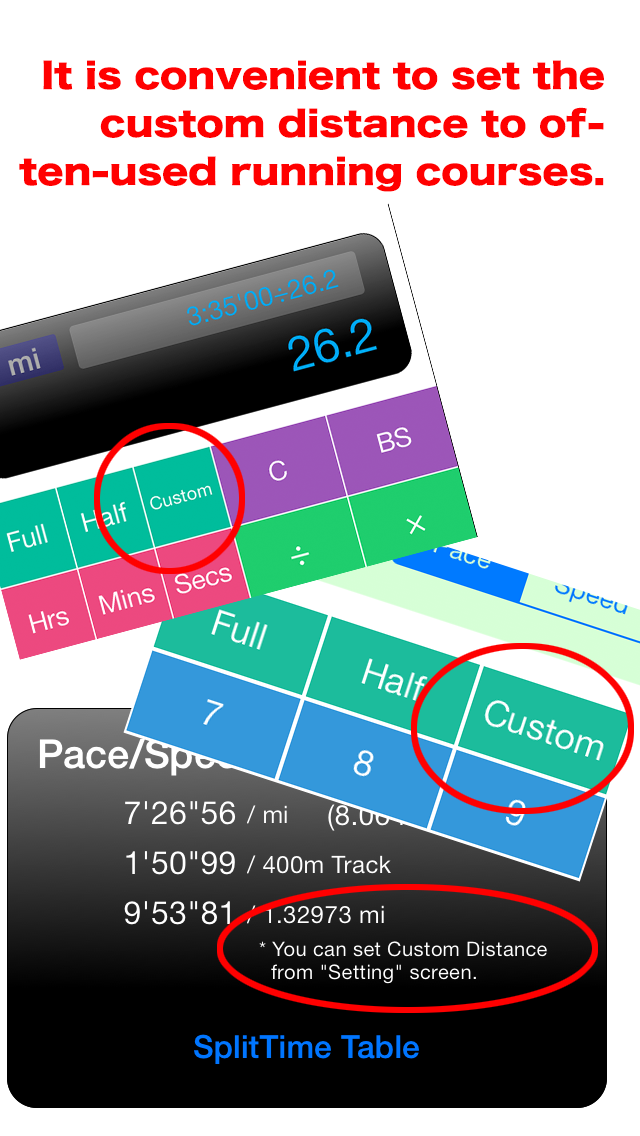FOR ALL RUNNERS

#Calculator For Runner

## News

• 2017/04/12 Ver2.2(iOS) / Ver1.1(Android) Update.
• 2015/11/08 Android App Release!!(Play Store)
• 2014/03/31 Ver2.0 Update(Mejor VerUp!)
• 2011/04/21 Ver1.1 Update(Bug Fix)

## What is "Calculator For Runner (CalcForRun)" ?

This is a calculator that allows all runners to simply calculate their lap-time and pace.
It feels the same to use as a typical calculator, but it can also carry out for different operations for calculating time.
Furthermore, it also has a special screen used to calculate a runner's pace prepared below.

• Judging by the distance(km or miles) traveled and time elapsed, the calculator can determine the pace and speed of a runner.
• Judging by the pace (or speed) and distance(km or miles) traveled by a runner, the calculator can determine the time elapsed.
• Judging by the pace (or speed) and time elapsed, the calculator can determine the distance(km or miles) traveled.

## What's New in Ver2.2(iOS) / Ver1.1(Android)

• Track mode has now been added to split time tables
On occasions where distance equals 800m(0.8km)/1000m(1km)/1500m(1.5km)/2000m(2km)/5000m(5km)/10000m(10km), split time tables can now be displayed on the basis of track distance, instead of 1km intervals.

• A search function has been added for crossing times at cheering points
A mode has been added to estimate cheer point crossing times, calculated from estimated finish times or crossing times for the preceding lap point.

## What's New in Ver2.0

• The calculator is compatible with four inch displays and the iOS7 screen.
• The user can change the settings from full-marathon, half-marathon, or a user-determined distance with only one touch.
• The pace calculating screen can also be used to show split-times.
• Using the backspace button, the user can return to their entries prior to their current one.## Calculation Rule

• Measuring time requires choosing seconds, minutes or hours as the lowest unit of calculation.
• The numerical value of time for addition will be calculated using seconds.
• In the case that numerical values are subtracted from time, calculations will be done using seconds.
• Numerical values cannot be subtracted from time.
• When the numerical value is divided by time, speed is calculated.
• 「The multiplication of numbers and time will become time.
However, in the case that the previous calculation's result was speed, the numerical value of distance shall be calculated.
• Time cannot be multiplied by Time.

## Contact

Any flaws, defects, demands, or feedback should be sent to us via e-mail(iphonesupport@dot-whim.com）.